# 第一模块：开发基础 第1章·Python基础语法

## 编程语言介绍

机器语言：

由于计算机内部只能接受二进制代码，因此，用二进制代码0和1描述的指令称为机器指令，全部机器指令的集合构成计算机的机器语言，机器语言属于低级语言。

优缺点：优点是最底层，速度最快；缺点是最复杂，开发效率低。

汇编语言：

汇编语言的实质和机器语言是相同的，都是直接对硬件操作，只不过指令采用了英文缩写的标识符，更容易识别和记忆。

优缺点：优点是比较底层，速度最快；缺点是复杂，开发效率低。

高级语言：

高级语言是大多数编程者的选择，和汇编语言相比，它不但将许多相关的机器指令合成为单条指令，并且去掉了与具体操作有关但与完成工作无关的细节，这样就大大简化了程序中的指令。

高级语言主要是相对于汇编语言而言，它并不是指某一种具体的语言，而是包括了很多编程语言，如C\C++、Java、PHP、Python、GO、C#等都是属于高级语言。

高级语言分为两类：编译型、解释型

编译型语言如：C、C++

解释型语言如：Python、Java

优缺点（编译型）：优点是执行速度快，不依赖语言环境运行；缺点是跨平台差。

优缺点（解释型）：优点是跨平台好，一份代码，到处使用；缺点是执行速度慢，依赖解释器运行。

## Python发展史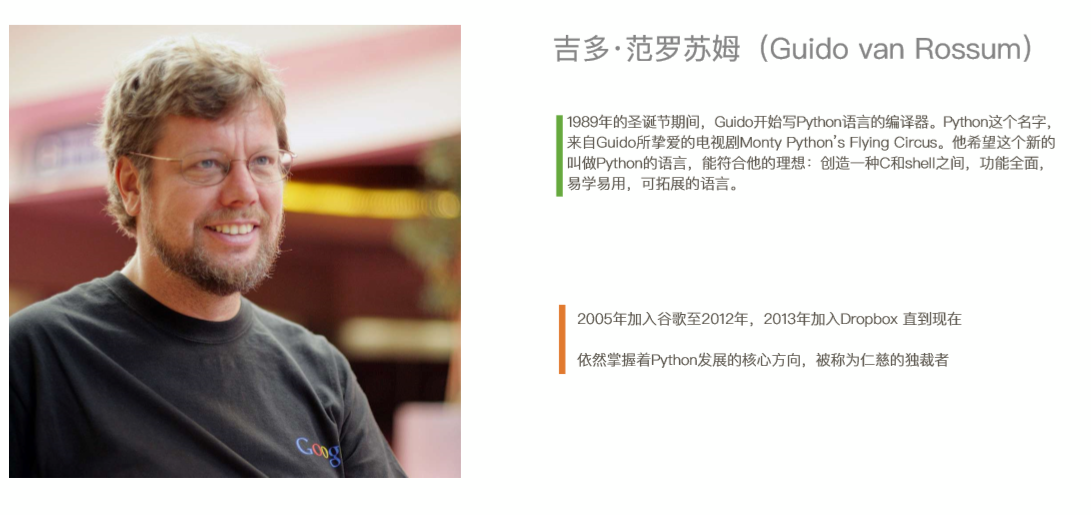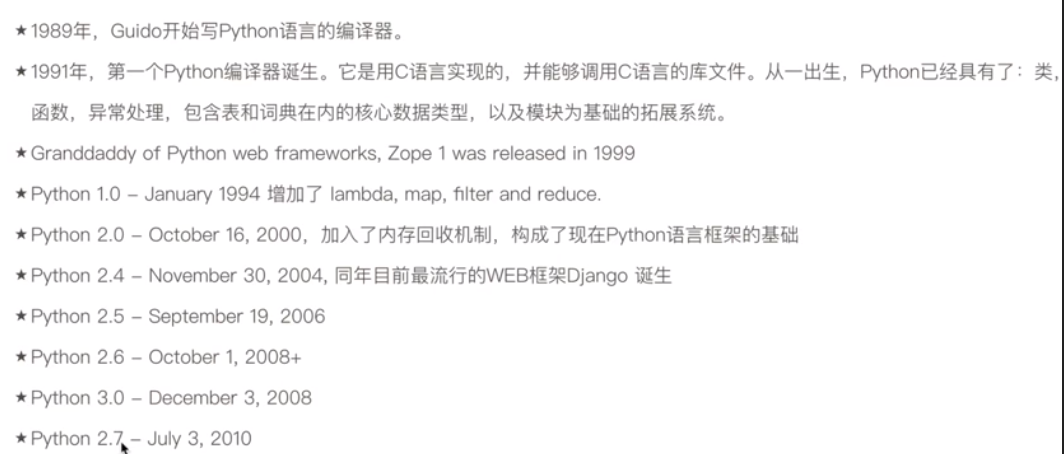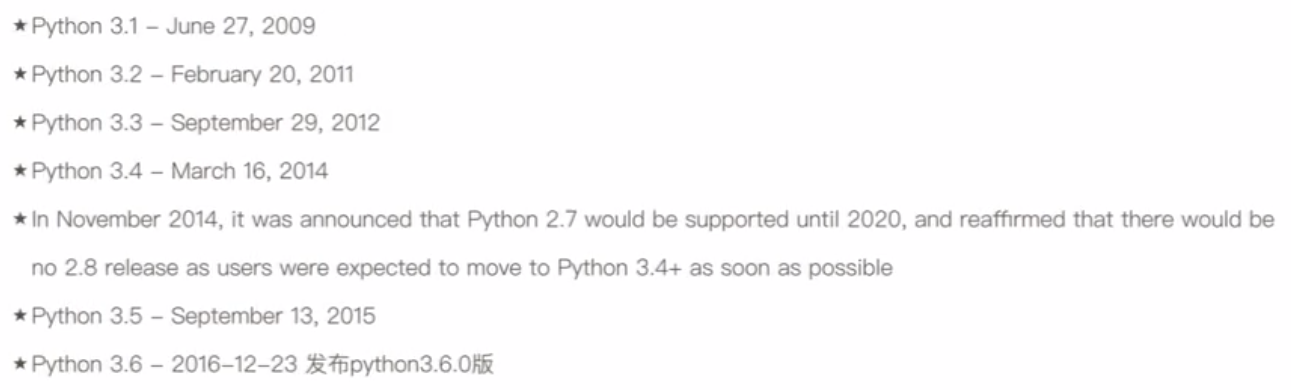## Python解释器类型 & Python2or3

Python解释器有以下几种：

CPython：最常用的python解释器，从官网下载也是CPython，因为使用C语言写的所以叫做CPython。

IPython：

PyPy：

Jython：

IronPython：

## 变量

name = "Alex"

age = 20

Variables are used to store information to be referenced and manipulated in a computer program. They also provide a way of labeling data with a descriptive name, so our programs can be understood more clearly by the reader and ourselves. It is helpful to think of variables as containers that hold information. Their sole purpose is to label and store data in memory. This data can then be used throughout your program.

python里的关键字不能设为变量名，如：and, as, pass, print 等等

AgeOfOldboy = 23

NumberOfStudents = 60

age_of_oldboy = 23

number_of_students = 60

a = 1

b = a

a = 3

AGE_OF_OLDBOY = 56

## 用户交互

name = input("please input your name:")
password = input("please input your password:")

## 注释

name = input("please input your name:")

'''

'''
print("Hello world.")

"""

"""
print("Hello world.")

## 数据类型：

Python 常用的数据类型有如下：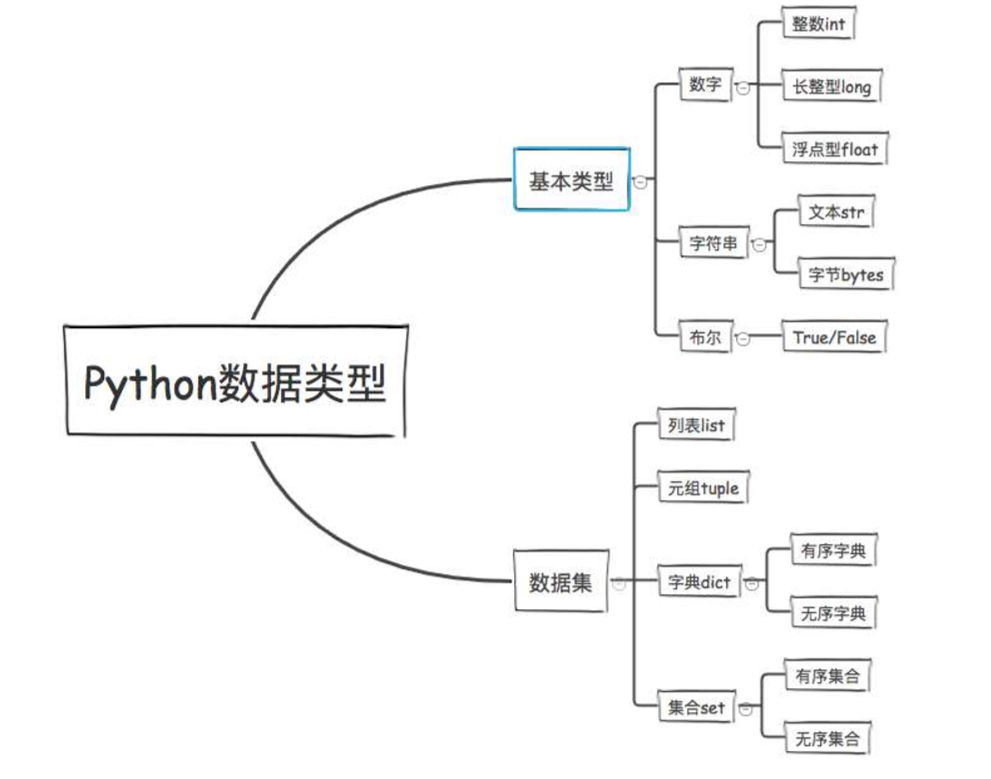int（整型）

long（长整型）

### 字符串类型：

name = "Alex"  # 双引号
age = "22"  # 数字加上引号也是字符串
age = 22  # 数字 int
msg = ''' My name is Alex '''  # 多引号也是字符串
hometown = 'Sichuan'  # 单引号

msg = "My name is Alex, I'm 22 years old."   # I'm中间有单引号出现

msg = '''

'''

>>> name = "Alex"
>>> age = "22"
>>> name + age
'Alex22'
>>> name * 10
'AlexAlexAlexAlexAlexAlexAlexAlexAlexAlex'
>>>

### 布尔类型

>>> a = 3
>>> b = 5
>>> a > b
False
>>> a < b
True
>>>

a = 3
b = 5
if a > b:
print("a is bigger than b.")
else:
print("a is smaller than b.")

### 格式化输出：

name = input("Name:")
age = input("Age:")
job = input("Job:")
hometown = input("Hometown:")

info = '''
--------------- info of %s -------------------
Name:          %s
Age:           %s
Job:           %s
Hometown:      %s
-------------------end-----------------------
''' % (name, name, age, job, hometown)
print(info)

Name:Alex
Age:23
Job:Works
Hometown:Sichaun

--------------- info of Alex -------------------
Name:          Alex
Age:           23
Job:           Works
Hometown:      Sichaun
-------------------end-----------------------

%s 表示string

%d 表示digit

%f 表示float

age = int("22")    # 将字符串22转换为数字的22

## 运算符% 取模，可以用来判断奇偶数，比如上面这个表格。python2里 != 和 <> 是一样的，python3里没有 <>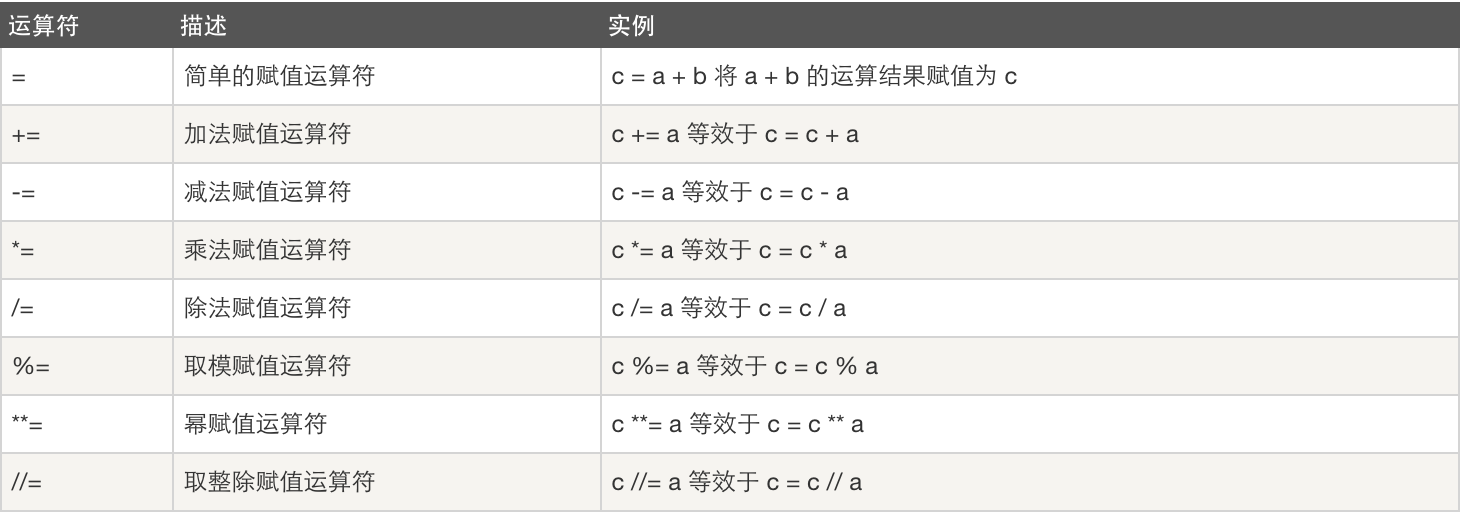逻辑运算：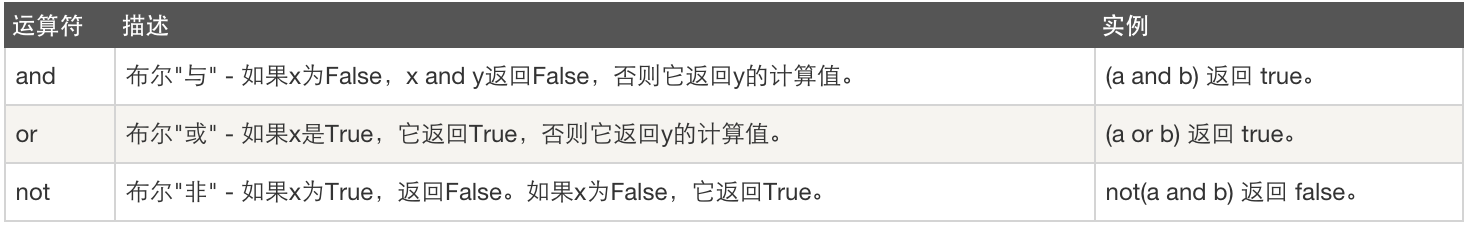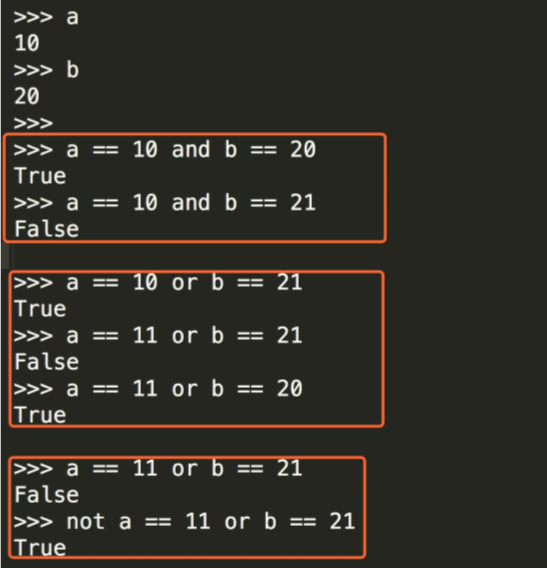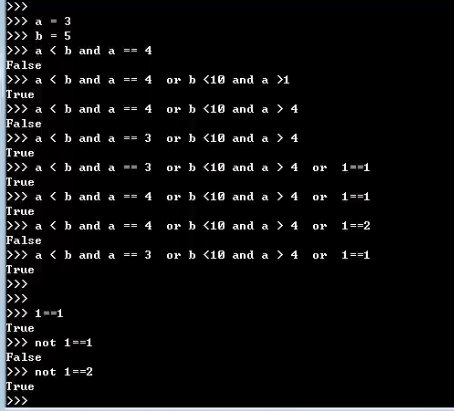### 流程控制

if 条件:
满足条件后要执行的代码

age = 56
if age > 50:
print("你的年龄大于了50岁.")

if 条件:
满足条件执行代码
else:
if条件不满足就执行这段代码

age = 30
if age > 50:
print("你的年龄大于了50岁.")
else:
print("可以，你还可以折腾几年.")

name = input("Name:")
sex = input("Sex:")
if sex == "female":
print("我喜欢女生")
else:
print("一起来搞基")

name = input("Name:")
sex = input("Sex:")
age = int(input("Age:"))
if sex == "f":
if age < 28:
print("我喜欢女生")
else:
print("姐弟恋也挺好哦")

name = input("Name:")
sex = input("Sex:")
age = int(input("Age:"))
if sex == "f":
if age < 28:
print("我喜欢女生")
else:
print("姐弟恋也挺好哦")
else:
print("一起来搞基")

if 条件:
满足条件执行代码
elif:
上面的条件不满足就走这个
elif:
上面的条件不满足就走这个
elif:
上面的条件不满足就走这个
else:
上面所有的条件不满足就走这个

练习1：猜年龄

age = 25
guess_age = int(input("please inpout guess age:"))
if guess_age > age:
elif guess_age < age:
else:
print("good, you got it.")

A　　90-100

B　　80-89

C　　60-79

D　　40-59

E　　0-39

score = int(input("please inout your score:"))
if score > 100:
print("分数最多只有100分。")
elif score >= 90:
print("A")
elif score >= 80:
print("B")
elif score >= 60:
print("C")
elif score >= 40:
print("D")
elif score >= 0:
print("E")
else:
print("分数没有负数")

### While循环

while 条件:
执行代码...

count = 0
while count <= 100:
print("loop", count)
count += 1

count = 0
while count <= 100:
if count % 2 == 0:
print("loop", count)
count += 1

count = 0
while count <= 100:
if count == 50:
pass
elif count >= 60 and count <= 80:
print(count * count)
else:
print("loop " , count)
count += 1

age = 25
count = 0
while count < 3:
if guess_age > age:
elif guess_age < age:
else:
print("good, you got it.")
count += 1

while是只要后边条件成立（也就是条件结果为真）就一直执行，怎么让条件一直成立呢？

count = 0
while True:
print("你是风儿我是沙，缠缠绵绵到天涯...", count)
count += 1

#### continue vs break

break 用于完全结束一个循环，跳出循环体执行循环后面的语句

continue 和 break 有点类似，区别在于continue只是终止本次循环，接着还执行后面的循环，break则完全终止循环。

break：

count = 0
while count <= 100:
print("loop", count)
if count == 5:
break
count += 1
print("---- out of loop ----")

loop 0
loop 1
loop 2
loop 3
loop 4
loop 5
---- out of loop ----

continue语句：

count = 0
while count <= 100:
count += 1
if count > 3 and count < 97:
continue
print("loop", count)
print("---- out of loop ----")

loop 1
loop 2
loop 3
loop 97
loop 98
loop 99
loop 100
loop 101
---- out of loop ----

age = 25
count = 0
while count < 3:
if guess_age > age:
elif guess_age < age:
else:
print("good, you got it.")
break
count += 1

count = 0
age = 26
while count < 3:
if user_guess == age:
print("恭喜你答对了。")
break
elif user_guess < age:
else:
count += 1
if count == 3:
choice = input("还想继续吗？(y/n)")
if choice == "y":
count = 0

age = 25
count = 0
while True:
if count == 3:
res = input("do you want to continue? (y/n):")
if res == "y":
count = 0
continue
else:
print("bye bye.")
break
if guess_age > age:
elif guess_age < age:
else:
print("good, you got it.")
break
count += 1

#### while ... else

while后面的else作用是指，当while循环正常执行完，中间没有break中止的话，就会执行else后面的语句

count = 0
while count <= 5:
count += 1
print("loop", count)
else:
print("循环已经正常执行完毕。")
print("----  out of while loop ----")

loop 1
loop 2
loop 3
loop 4
loop 5
loop 6

----  out of while loop ----

posted @ 2018-04-28 12:14  alexchenx  阅读(209)  评论(0编辑  收藏